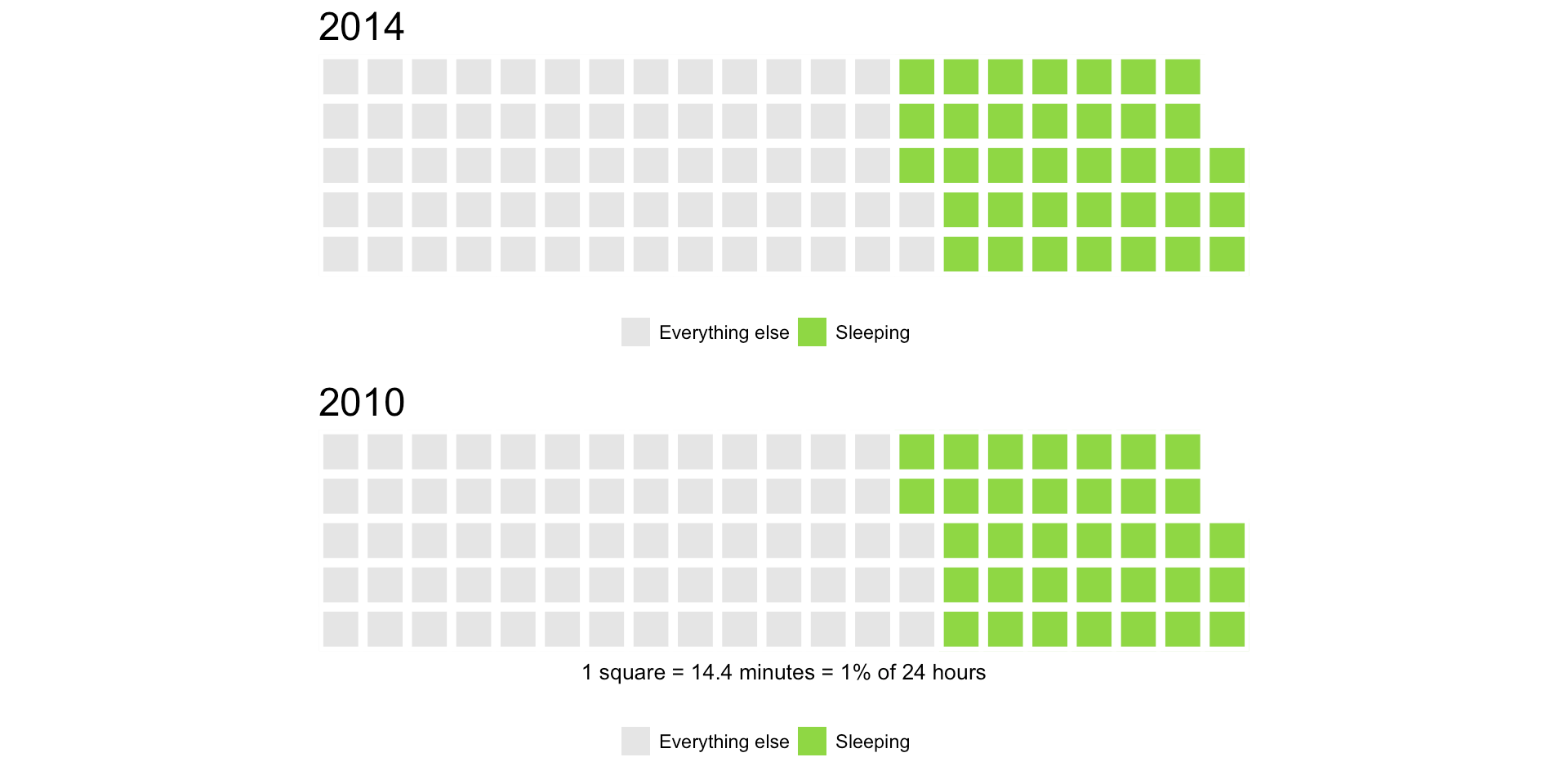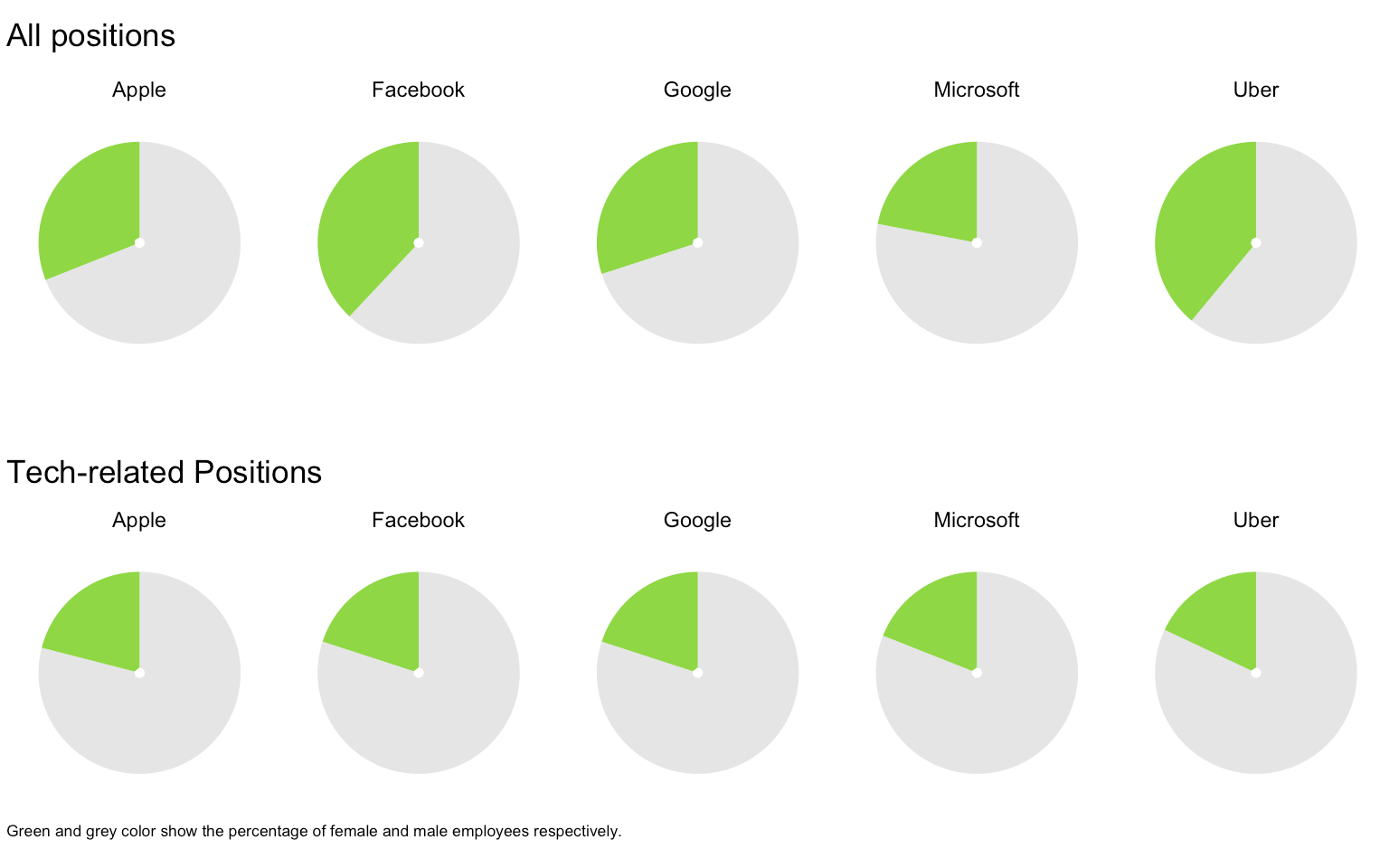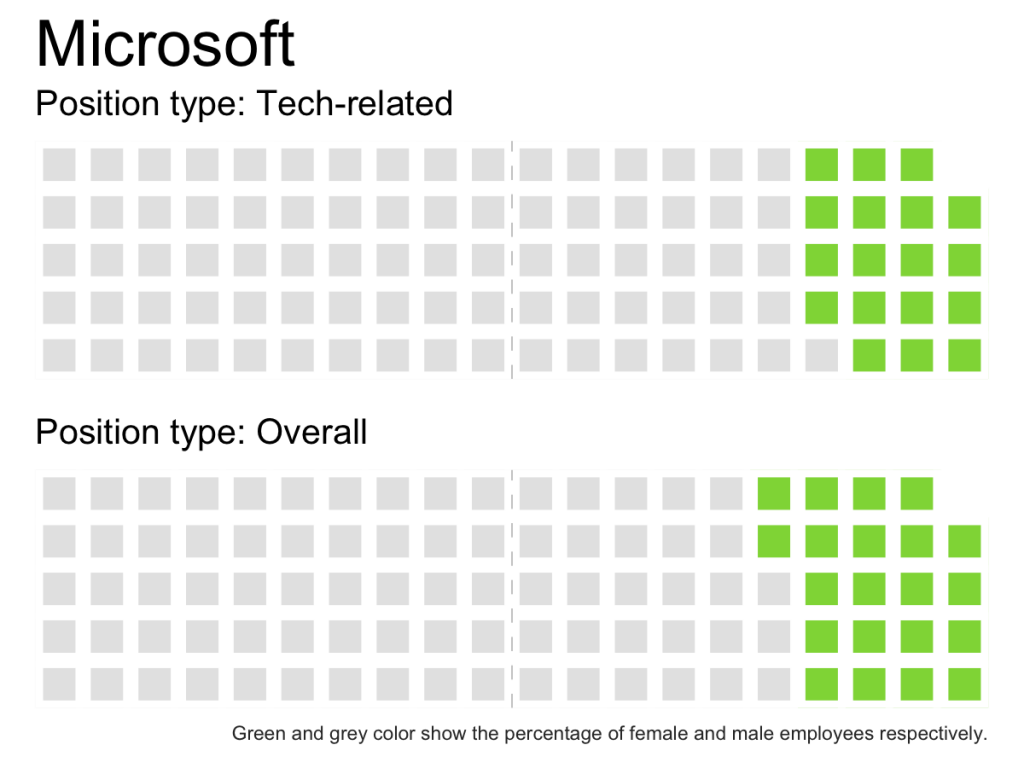Want to share your content on R-bloggers? click here if you have a blog, or here if you don't.

# Parts-to-Whole Charts

Creating good charts is difficult.

Parts-to-whole charts are even more difficult to create, not technically, but to generate a higher level of efficacy. The most common parts-to-whole chart, in which you show different proportions of a measure, is a pie chart. So much so that a hotel advertised its meeting rooms with this poster:Another parts-to-whole chart is a stacked-bar graph.

Pie charts and stacked bar graphs share similar challenges: hard to distinguish parts. This happens when you have tiny or too many proportions. And just when you think you’re helping the problem by adding colors to distinguish the parts, the problems become even worse.

So what to do when you must show the parts?

There are a few alternatives, not without their own problems:

• Treemap
• Waffle chart or square area graph

I recently saw Cole Nussbaumer Knaflic’s challenge on her blog to create a waffle chart or square area graph. (If you have not read her book Storytelling with data, buy a copy now. I had been thinking about writing a post about this topic, so this was timely.

Let’s look at creating waffle charts using the two graphics I saw in the Wall Street Journal and in the Economist. We can improve both of these charts.

## Not a short stack of waffles

The Wall Street Journal published this story on how an average American spends his or her day.

They used a big, really big, waffle chart to show these activities in the form a working women, who plays soccer and drinks martinis. This infographics falls prey to focusing on the cuteness of the graphic rather than just giving the numbers a.k.a. “interesting”.

It is a good thing that we sleep for most of time, otherwise, I don’t know how the designer would have gotten away without using the other body parts to describe the activities. They were successful in using some relevant body parts for some of the activities. For example, the brain for education, a hand with a briefcase for work, a leg for sports. But not every activity was that lucky. For example, a knee for religious activities and a foot for caring for household members.After a long day of taking care of the kids when they say “ungrateful” things, I’m sure that’s exactly how my wife feels, “taking care of the household members, my foot!” So, maybe, there’s some logic to this…A good balance

Anyway!!

Can we create some charts that give the same information with less ink, thus improving the data to ink ratio?

I tried a few things.

First, I tried creating a waffle chart, using the waffle library in R and following these steps.

BTW, rather than showing the total number of minutes for each activity, I thought showing the percentage of the day that an activity takes would be useful.

```library(ggplot2) # for plotting
library(dplyr) # for data manipulation
library(waffle) # to create waffle charts
library(viridis) # for pretty and sensible colors

library(showtext) # to change the font type

#font_add("Opensans", regular =  "OpenSans-Regular.ttf", bold = "OpenSans-Bold.ttf")
showtext_auto()```

```wsj_activity <- read_csv("day-in-life-wsj.csv")

yr_14_act <- setNames(subset(wsj_activity, Year == 2014)\$percentageofday,
subset(wsj_activity, Year == 2014)\$Activity)

yr04_act <- setNames(subset(wsj_activity, Year == 2004)\$percentageofday,
subset(wsj_activity, Year == 2004)\$Activity)```

### Plot the data

```w1 <- waffle(parts = sort(yr_14_act*100, decreasing = TRUE),
rows = 5,
colors = viridis(n = 14, option = 'cividis'),
title = '2014',
legend_pos = "bottom")

w2 <- waffle(parts = sort(yr04_act*100, decreasing = TRUE),
rows = 5,
colors = viridis(n = 14, option = 'cividis'),
title = '2010',
xlab = '1 square = 14.4 minutes = 1% of 24 hours',
legend_pos = "bottom")

iron(w1, w2)```Huh?The legend box is bigger than the plot and you can’t differentiate any categories. At least the original graph succeeded in that aspect.

How to solve this problem?

We could try to highlight only one category or two categories combined. For example, it “seems” like we spend a lot of time on leisure and watching TV. How does that look when we plot that data?

```relaxing <- wsj_activity %>%
mutate(Activity = ifelse(Activity %in% c('Watching TV', 'Leisure and sports (excludes TV)'),
'Relaxing',
'Everything else')) %>%
group_by(Year, Activity) %>%
summarize(percentageofday = sum(minutes)/1440)

yr_14_act <- setNames(subset(relaxing, Year == 2014)\$percentageofday,
subset(relaxing, Year == 2014)\$Activity)

yr04_act <- setNames(subset(relaxing, Year == 2004)\$percentageofday,
subset(relaxing, Year == 2004)\$Activity)

w1 <- waffle(parts = sort(yr_14_act*100, decreasing = TRUE),
rows = 5,
colors = c('grey90', '#8FD744FF'),
title = '2014',
legend_pos = "bottom")

w2 <- waffle(parts = sort(yr04_act*100, decreasing = TRUE),
rows = 5,
colors = c('grey90', '#8FD744FF'),
title = '2010',
xlab = '1 square = 14.4 minutes = 1% of 24 hours',
legend_pos = "bottom")

iron(w1, w2)``````sleeping <- wsj_activity %>%
mutate(Activity = ifelse(Activity  == 'Sleeping',
'Sleeping',
'Everything else')) %>%
group_by(Year, Activity) %>%
summarize(percentageofday = sum(minutes)/1440)

yr_14_act <- setNames(subset(sleeping, Year == 2014)\$percentageofday,
subset(sleeping, Year == 2014)\$Activity)

yr04_act <- setNames(subset(sleeping, Year == 2004)\$percentageofday,
subset(sleeping, Year == 2004)\$Activity)

w1 <- waffle(parts = sort(yr_14_act*100, decreasing = TRUE),
rows = 5,
colors = c('grey90', '#8FD744FF'),
title = '2014',
legend_pos = "bottom")

w2 <- waffle(parts = sort(yr04_act*100, decreasing = TRUE),
rows = 5,
colors = c('grey90', '#8FD744FF'),
title = '2010',
xlab = '1 square = 14.4 minutes = 1% of 24 hours',
legend_pos = "bottom")
iron(w1, w2)```You could use this approach to either identify the biggest time consuming categories or the least. For example, how much time do we spend on education?

```education <- wsj_activity %>%
mutate(Activity = ifelse(Activity  == 'Education',
'Education',
'Everything else')) %>%
group_by(Year, Activity) %>%
summarize(percentageofday = sum(minutes)/1440)

yr_14_act <- setNames(subset(education, Year == 2014)\$percentageofday,
subset(education, Year == 2014)\$Activity)

yr04_act <- setNames(subset(education, Year == 2004)\$percentageofday,
subset(education, Year == 2004)\$Activity)

w1 <- waffle(parts = sort(yr_14_act*100, decreasing = TRUE),
rows = 5,
colors = c('grey90', '#8FD744FF'),
title = '2014',
legend_pos = "bottom")

w2 <- waffle(parts = sort(yr04_act*100, decreasing = TRUE),
rows = 5,
colors = c('grey90', '#8FD744FF'),
title = '2010',
xlab = '1 square = 14.4 minutes = 1% of 24 hours',
legend_pos = "bottom")
iron(w1, w2)```Ouch! Not much.This approach could work, but we can’t see all categories at once and it’s hard to spot the difference between years.

Which chart could work? That’s right. A horizontal, sorted bar chart!

```library(scales)
ggplot(data =  wsj_activity, aes(x = reorder(Activity, percentageofday, FUN=max), y = percentageofday, fill = factor(Year))) +
geom_bar(stat = "identity", position = "dodge") +
scale_y_continuous(labels = percent, limits = c(0, 0.4), breaks = seq(from = 0, to = 0.4, by = 0.1)) +
geom_hline(yintercept = seq(from = 0.1, to = 0.4, by = 0.1), color = "grey98") +
scale_fill_manual(values = c('grey90', '#8FD744FF'), name = "Year") +
theme_bw(base_size = 14) +
coord_flip() +
theme(panel.border = element_blank(),
panel.grid = element_blank(),
legend.position = "bottom",
axis.ticks.y = element_blank(),
axis.title = element_blank())```Or, we can try a dot chart:

```ggplot(data =  wsj_activity, aes(x = reorder(Activity, percentageofday, FUN=max), y = percentageofday, color = factor(Year))) +
geom_point(alpha = 0.95, size = 4) +
scale_y_continuous(labels = percent, limits = c(0, 0.4), breaks = seq(from = 0, to = 0.4, by = 0.1)) +
geom_hline(yintercept = seq(from = 0.1, to = 0.4, by = 0.1), color = "grey98") +
scale_color_manual(values = c('grey90', '#8FD744FF'), name = "Year") +
theme_bw(base_size = 14) +
coord_flip() +
theme(panel.border = element_blank(),
panel.grid = element_blank(),
legend.position = "bottom",
axis.ticks.y = element_blank(),
axis.title = element_blank())```What do you think? Would you still go for the balancing lady infographic, or would you use a sorted bar chart?

# William Playfair or: How I Learned to Stop Worrying and Love the Pie Chart

As we saw earlier, pie charts have tremendous recognition in the business world, and at the same time, they are often ineffective as a data visualization. I’ve had my preachy moments of “don’t use the pie chart, ever!” But there are instances in which pie chart is a better choice over other charts. Yes, I said it.

For example, the Economist, which usually publishes high-quality charts, published an article following Google’s firing of James Damore. This article discussed gender diversity at tech firms. It included this graph, ironically, a dot chart:At first, it seems like a nicely designed chart. But when you look at it carefully, you observe that this dot chart hides the small proportion of female employees, especially in technological roles, at these companies. A pie chart, yes, a pie chart would show the proportions more clearly and draw the reader’s attention to the smaller number–though we won’t show the exact number, because that’s irrelevant as long as we can make our readers reflect on the matter at hand, the gender diversity problem.

```library(tidyr)
library(gridExtra)
gender_pct <- data.frame(type = c(rep('Overall', 5), rep('Tech-related', 5)),
male_pct = c(.61, .78, .62, .7, .69, .82, .81, .8, .8, .79 ))

gender_pct_both <- mutate(gender_pct, female_pct = 1 - male_pct) %>%
gather(key = gender, value = pct, -type, -company)

g1 <- ggplot(data = filter(gender_pct_both, type == 'Overall'),
aes(x = "", y = pct, fill = gender)) +
geom_col(position = position_fill()) +
scale_y_continuous(limits = c(0, 1), position = 'top', label = percent) +
facet_wrap(~company, ncol = 5, nrow = 2)
g1 <- g1 + coord_polar("y", start = 0) +
labs(title = "All positions", caption = "")
g1 <- g1 + theme_void() +
theme(legend.position = "none") +
scale_fill_manual(values = c("male_pct" = "grey90", "female_pct" = "#8FD744FF"))

g2 <- ggplot(data = filter(gender_pct_both, type == 'Tech-related'),
aes(x = "", y = pct, fill = gender)) +
geom_col(position = position_fill()) +
scale_y_continuous(limits = c(0, 1), position = 'top', label = percent) +
facet_wrap(~company, ncol = 5, nrow = 2)
g2 <- g2 + coord_polar("y", start = 0) +
labs(title = "Tech-related Positions",
caption = "Green and grey color show the percentage of female and male employees respectively.")
g2 <- g2 + theme_void() +
theme(legend.position = "none",
plot.caption = element_text(size = rel(0.6), hjust = 0)) +
scale_fill_manual(values = c("male_pct" = "grey90", "female_pct" = "#8FD744FF"))

grid.arrange(g1, g2)```Can you see the small percentage of female tech employees compared to all positions at Uber? Would you observe the same thing from the Economist’s dot chart?

I’m pretty happy with this graphic (though I could use more pleasing colors), but how would this data look using a waffle chart?

```create_waffle_by_company_type <- function(type, company) {
df <- filter(gender_pct_both, type == !!type & company == !!company)
gender_dist <- setNames(
df\$pct,
df\$gender)
w <- waffle(parts = sort(gender_dist*100, decreasing = TRUE),
rows = 5,
colors = c('grey90', '#8FD744FF'),
legend_pos = "none")
w <- w +
geom_vline(xintercept = 10.5,
size = rel(0.15),
linetype = 2,
color = "grey70") +
theme(axis.title.x = element_text(hjust = 0))
return(w)
}
create_waffle_by_company_type(type = 'Tech-related', company = 'Uber')

combine_waffles <- function(company){
w1 <- create_waffle_by_company_type(type = 'Tech-related', company = company) +
ggtitle(company, subtitle = "Position type: Tech-related")
w2 <- create_waffle_by_company_type(type = 'Overall', company = company) +
labs(
subtitle = "Position type: Overall",
caption = "Green and grey color show the percentage of female and male employees respectively.") +
theme(plot.caption = element_text(color = "grey20", size = rel(0.5)))
iron(w1, w2)
}```

I created a couple of helper functions and then ran those on every company:

```sapply(unique(gender_pct_both\$company), function(company){
ggsave(filename = paste0(company, 'waffle.png'),
plot = combine_waffles(company),
width = 4,
height = 3)
})```Which one do you think are more effective: pie charts or waffle charts?

You saw a gigantic waffle chart that can be turned into a dot chart for higher effectiveness. And, you saw an ineffective dot chart turned into pie charts. What does that tell you? That I’m confusing? Maybe. Or, that every chart has its place and you as its designer must think about its objectives to create the right graphic.